Surface Area Definition, Formulas & Worksheet!

## Learn everything from definition of Surface Area to formulas:

### What is Surface Area?

Surface area is defined as the surface size of any object. We have different shapes with different surface areas.

### What is Area?

Area is defined as the size of any object. Area is measured in square units. Some of the units of area are centimeter square, meter square, feet square, inches square, etc.

### What is Lateral Surface Area?

Lateral surface area is defined as the side surface area of an object.

## What are the formulas to calculate volume of common shapes around us:

Square:- Square is a shape which has four equal sides and all the angles are right angles as shown in figure below.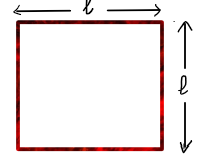The area of square is given by formula:-

Area =  length × length

Rectangle:-Rectangle is a shape which has four equal and opposite sides and all the angle are right angles.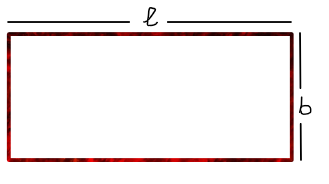The area of rectangle is given by formula:-

Triangle:-  Triangle is a shape having three sides.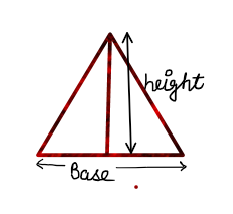The area of triangle is given by the formula:

Area =  12 (base × height)

Circle:- Area of circle can be given as under:-

Area = πr^2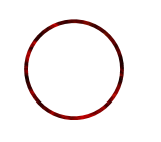Cylinder:- The area of square is given by formula:-

Total Surface Area =  2πr(r+h)

Lateral Surface area = 2πrh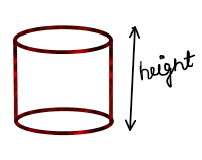Cone:-  Area of cone is given by the formula:-

Total Surface Area= r+h^2+r^2

Lateral Surface Area = rh^2+r^2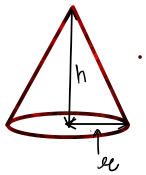^
Sphere:- Area of sphere is given by:-

Area = 4πr^2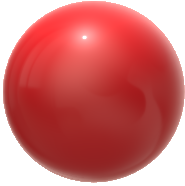## Surface Area Questions & Answers - Sample

Que 1:- What is the area of the square having sides 3m ?

Ans   Length of side of square = 3 m

Area of square =  length × length

= 3 × 3 = 9 m^2

Que 2 :- What is the radius of the sphere having area 16 m2?

Ans  Area of sphere = 16 m^2

As area of sphere = 4πr^2

4πr^2=  16                         ^

r^2= 4

r = 2 m

Thus, the radius of the sphere is 2m

Que 3:- What is the lateral and total surface area of the cylinder having height  4cm and radius 2 cm ?

Ans  Height of cylinder , h = 4 cm

Radius of cylinder , r = 2 cm

Total surface of area =  2πr(r+h) = 2π^2 (2+4)

= 24πcm^2

## Say no to Surface Area Homework Stress and Yes to A+ Grades

The Concept of surface area was introduced to students around the United States during the middle school years. Students are taught how to calculate and solve problems related to any three-dimensional geometrical shapes.

Just like the topic of volume, students learning the details about three dimensional shapes deal with complex topics like different shapes have different surface areas.

The topic of surface area can be many times confused with the volume and hence get a student confused. Therefore it is essential to get the correct guidance so that students from the very early on understand the differences between the two topics as well as feel confident solving homework questions or even attempting surface area questions in their exams.

Surface area is one of those concepts that has a lot of real life application in our day to day life. One needs surface area to figure out the amount of frosting needed for a birthday cake, we can use surface area of a cylinder to understand the amount of paint needed for a cylindrical fuel tank, we can even find applications of surface area and its formulas in marketing role such as based on the dimensions of the object how big should the label be for the box.

We have designed our help to meet study needs of students around the clock. We have some of the best math experts to help your child with the  core concepts of surface area.

Available 24/7, our tutors can handle all surface area related questions and get you the correct solutions at the most reasonable prices.

## Follow these steps to available homework help at TutorEye:

Step 1: Fill out the help homework form & post your homework questions.

Step 2: Choose from the multiple request that fit your budget and timeline.

Our work is 100% original, and we stand behind the quality of support and guidance provided. Our tutors help hundreds of kids daily to understand difficult concepts, find solutions and improve Math grades.

## Surface Area Frequently Asked Questions

Question 1: What is the formula for surface area?

The surface area of any object is the space occupied by that object. Let us take an example of a rectangular figure whose length and breadth are given. To calculate the area of this rectangular figure we need to use a formula and multiply its length by the breadth. The unit of surface area is square units, in the previous case if the length is in meters and breadth is also in meters then the unit of area is square units. Different shapes have different areas and we have formulas to calculate the surface area of each object.

 SHAPE TOTAL SURFACE AREA Cube 6 (side)2 Sphere 4 π× (radius)2 Cylinder 2π × (radius + height) Cuboid 2 (lb+ bh + hl) Prism {(Perimeter of the base) × height} + 2 ×Base Area Hemisphere 3π × (radius)2 Cone π × radius × (slant height + radius)

Question 2: What is the easiest way to find surface area?

The easiest way to find the surface area of any figure is to use the formula of surface area for that figure. The total surface area of the figure is the total space occupied by the figure, if you want to paint a wall the surface area is the entire region where paint will be applied. For a three dimensional figure the total surface area is equal to the sum of the areas of all the surfaces. Consider a cube having side equals to s, we know that it has a total six surfaces one is in the front, other is at the back, one is at the top, other is at the bottom, and one is on the left and other on the right. The area of one of the surfaces of the cube is (side)2, hence the surface area of the six faces is 6 × (side)2 which is equal to the total surface area of the cube.

Question 3: What is the surface area of a rectangle?
Rectangle is the two dimensional figure with four sides and four right angles, the opposite sides of rectangle are equal and all angles are of 900. The longer side of the rectangle is called its length and the shorter one is the breadth, the area of the rectangle is equal to length times its breadth it is the space occupied by the rectangle. We can consider a square as one of the types of the rectangle with its all sides equal to each other and all angles equal to ninety degrees. Hence the area of the square is equal to side times side or (side)2.

Question 4: What is the surface area of a prism?
A polyhedron figure with two parallel polygonal bases is called a prism, these two polygonal bases are connected through the lateral surface.  The Surface area of any prism is equal to the sum of the area of the two polygonal bases and the area of the lateral surfaces. The common prisms known to us are rectangular prism, triangular prism, pentagonal prism and hexagonal prism. We can calculate the surface areas of these prisms using the same concepts: calculating the areas of two polygonal bases and lateral surfaces and finally adding them.

Question 5: How to find the surface area of a rectangular prism?
A rectangular prism is the three dimensional solid figure with six rectangular faces, these six faces are at 90 0 to each other and the opposite faces are congruent to each other. The surface area of the rectangular prism can be calculated by calculating the area of each six faces and adding them all together. The formula for the surface area of the rectangular prism is 2(bl + lh + hb) where l is equal to the length of the base of the rectangular prism, b is the width of the base and h is the height of the rectangular prism. A special case where all the faces of the rectangular prism are congruent, this type of prism is termed as a cube it has all its sides equal l=b=h = a, hence the surface area of this figure is equal to 6(a)2.

Question 6: How to find the surface area of a triangular prism?
A Triangular prism is a three dimensional figure having two triangular bases connected by the three rectangular faces. The surface area of the triangular prism is the sum of the areas of the two triangular bases and the area of the three rectangular faces. The area of the triangular base is 0.5× b ×h and the area of the rectangular face is b×h, hence the surface area of a triangular prism is given by the formula:  a×b  + 3 ×b×h , where b is the  base of the prism, a is the side and h is the height of the prism.

Question 7: How to find the surface area of a cylinder?
A three dimensional figure with the two circular bases parallel to each other joined by a curved surface. The surface area of the cylinder is equal to the sum of areas of the two circular bases and the curved part, if we open the curved part it gives a rectangle this area is known as curved surface area or lateral surface area.
The total area of the cylinder = Curved surface area +  Base area.
Curved surface area = 2×π×r×h where r is the radius of the circle of the base and h is the height of the cylinder
Base area = 2×π×r2
Total area of the cylinder = 2×π×r×h +2×π×r2

Question 8: How to find the surface area of a cube ?
The surface area of the cube is the total space occupied by the sides of the figure in the space. A cube has six square faces of equal side and to calculate the area of the cube we find out the area of all the faces. The face of the cube is square in shape hence the area of each face of the cube is (side)2, the cube has six faces hence the total surface area of the cube is equal to the sum of the areas of the six faces.
Total surface area of the cube = a2+ a2+ a2 +a2 +a2 +a2
= 6 a2

Question 9: How to find the surface area of a sphere?
The surface area of the sphere is the total outer space occupied by the sphere. Sphere is a round three dimensional figure like ball, globe, balloon etc. If we assume a cylinder of radius exactly equal to the radius of the sphere, then the surface area of the sphere is equal to the lateral surface area of the cylinder.
Total surface area of sphere = lateral surface area of the cylinder = 2×π×r×h
height of the cylinder = diameter of the radius
hence the total surface area of the sphere = 4×π×r2

Question 10: What is lateral surface area?

The lateral surface area of the three dimensional object is the area of the curved surface or the total area excluding the top and bottom areas. Let us take an example of cube if we want to calculate the lateral surface area of the cube, the cube contains six faces if we remove the top and bottom faces we get only four faces left, right, front and back, then the area of these four faces will be 4 (side)2.
Hence the lateral surface area of the cube is 4 (side)2
Similarly we can find out the lateral surface area of the cuboid will be 2(l+b)×h which can also be written as (perimeter of the base) × height.

Question 11: What is the surface area of a cylinder?
The surface area of the cylinder is the total space covered by the cylinder, the surface area of the cylinder is equal to the sum of the two circular base areas and curved surface area. The curved surface area is the space between the two parallel circular bases. If we open this curved figure it will form a rectangle of length 2×π×r and breadth of h hence the curved surface area is 2×π×r×h.
And the area of the two circular bases is 2×π×r2.
Hence the surface area of cylinder =  2×π×r×h + 2×π×r2
= 2 π ×r (h+r)